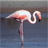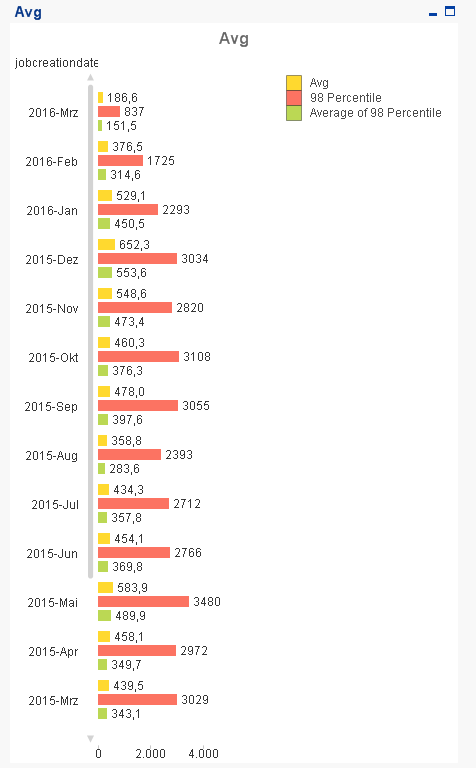# QlikView App Dev

Discussion Board for collaboration related to QlikView App Development.

Announcements
Our next Qlik Insider session will cover new key capabilities. Join us August 11th REGISTER TODAY
cancel
Showing results for
Did you mean:Creator II

## Average of fractile

Hi,

I want to calculate the average of all values below the 98th fractile.

In my example I have a counter (called impressions) and I want to calculate the average of all counters cutting off the top 2 percent.

I tried the expression

=Avg(If(impressions < Fractile(impressions,0.95),impressions))

But I receive the error "Allocated memory" exceeded. I do not know why.

Does someone have any idea how I could solve this?

I also tried to find a function which returns values which match a certain condition (in my case being lower than a fractile), so that I could use it for the average caluclation, but I wasn't successful.

Appreciate any hint!

Thanks, Nigel

1 Solution

Accepted SolutionsMVP

Try this:

=Avg(Aggr(If(impressions < Fractile(TOTAL <jobcreationdateJahrMonat> impressions,0.98), impressions), jobcreationdateJahrMonat, jobid))13 RepliesMVP

Try

=Avg(If(impressions < Fractile(TOTAL impressions,0.95),impressions))MVP

You might have to use Aggregation function here. When you are calculating the fractile, is it the over fractile or based on certain dimension?Creator II
Author

Actually I have one more dimension (Month). I've added a qvw. I used the calculation of swuehl =Avg(If(impressions < Fractile(TOTAL impressions,0.98),impressions)) but I think the result is not correct.MVP

What numbers are you expecting?MVP

Try

=Avg(Aggr( If(impressions < Fractile(TOTAL<Month> impressions,0.95),impressions), Month,impressions))MVP & LuminaryCreator II
Author

For example in March 2016, if the 98th fractile is 837, then I select the month March 2016 and all values <= 837, and the average calculates 151,7. Hence, when I remove the selections, I would expect the average of the 98th fractile in March 2016 to be 151,7.MVP

You may also need to adapt the 0.95 in the fractile() function to 0.98 if you are looking for 98th percentile.Creator II
Author

Hmm, sorry but I would expect the Average of 98th Percentile to be

151,5 in March 2016

314,4 in February 2016.

I tried both of your suggestions but do not get these values.Community Browser